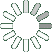Puzzle Command
Daily Puzzle
Number Logic #22
March 29, 2015Find a 6 digit number where:

The 1st digit is the number of 0s in the number.
The 2nd digit is the number of 5s in the number.
The 3rd digit is the number of 6s in the number.
The 4th digit is the number of 7s in the number.
The 5th digit is an odd number.
The 6th digit is the the number of 1s in the number.

All digts are lower than 4.

Puzzle Notes
6 digit numbers range from 100,000 to 999,999.
Enter in your solution below and click submit.